# Trucks

Truck take you the some amount of clay in 30 hours. Second truck in 40 hours. Trucks carted the clay away some time together then the second truck broke down and the first one completed the job in five hours. What time worked together?

Correct result:

x =  0 h

#### Solution:

Equation is non-linear.

## Calculation:

Equation is linear and have one solution:

7x-100=0

x=14.2857142857

Our simple equation calculator calculates it.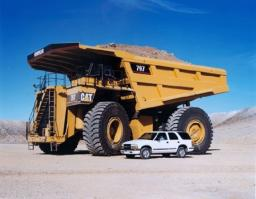We would be pleased if you find an error in the word problem, spelling mistakes, or inaccuracies and send it to us. Thank you!Tips to related online calculators
Do you have a linear equation or system of equations and looking for its solution? Or do you have quadratic equation?
Do you want to convert time units like minutes to seconds?

## Next similar math problems:

• Two workers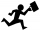One worker needs 40 hours to do a job, and the second would do it in 30 hours. They worked together for several hours, then the second was recalled, and the first completed the job itself in 5 hours. How many hours did they work together, and how much did
• Two workers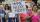The first worker completed the task by himself in 9 hours, the second in 15 hours. After two hours of joint work left first worker to a doctor and the second finished the job himself. How many hours worked second worker himself?
• The dam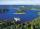The water reservoir is filled with first tributary for 25 hours second tributary for 35 hours. Some time were both inlets open, then a second tributary closed and the tank was filled in four hours. What time water flowed from both tributaries? (Expressed
• Welders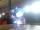Welder do the job for six hours, the second one for 8 hours. How long do the job third welder if all together can do job in 2 hours?
• Digging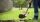The first worker would dig a trench in 6 hours. For the second, the same work would take 4 hours. How long did it take for the trench to be dug if they worked together?
• Tractors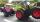Two tractors plow the field in 4 hours together. If the first tractor plow half of the field and then the second tractor completed the job, it would take 9 hours. How many hours does the field plow for each tractor separately?
• TurnersTwo turners perform joint work in 3 hours. The first turner would perform the job himself in 7 hours. How long would the same job last for a second turner if he worked alone?
• Three peopleThree people start doing a work at a same time. The first worked only 2 hours. The second ended 3 hours before the end. On an individual basis, it would take the first time to do the work 10 hours, second 12 hours and 15 hours third. How many hours did it
• WorkThe first worker would need less than 4 hours to complete the task than the other worker. In fact, both workers worked for two hours together, then the first worker did the remaining work himself. In what proportion should the remuneration of the workers
• Bricklayers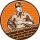The first bricklayer would build a wall in 3 days, the second in 4 days. The first bricklayer worked alone for a day, then the second bricklayer came to his aid and they finished the work together. How long did it take to build the wall?
• TruckIn 7 hours started from town Krnov truck at speed 40 km/h. Passenger car started against it in 8 hours 30 minutes from the city of Jihlava at speed 70 km/h. Distance between this two cities is 225 km. At what time and at what distance from Krnov this twoLucy, Tereza, and Petra decided to compile the compulsory reading records together. It would take 30 days for Lucia alone, 36 for Tereza and 45 for Petra. How long will it take to make records if they will work together?The first worker would himself completed the work in 8 hours, the other in 6 hours. After two hours of joint work, the worker left the first worker to a doctor, and others completed the work himself. How many hours worked the other worker himself?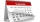The family ordered two companies to modify the garden. The first would be done in 20 days, the second would take 40% less time. How long will they work together if the second company started working when the first worked for 4 days?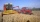The first harvester will harvest the grain from the field in 20 hours, the second, the more powerful in 10 hours. How long will take harvest this field by both harvesters, if the second harvester has to be set up first and this takes 2 hours? The first ha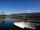The first pump fills the tank in 24 hours second pump in another 40 hours. For how long will it take to fill the tank if it operates both pumps at the same time?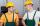Two workers work together and perform the job in 12 hours. If one has done half the work and then the second worker next half of the work it will take 25 hours. How long would it take job them separately?